Download Presentation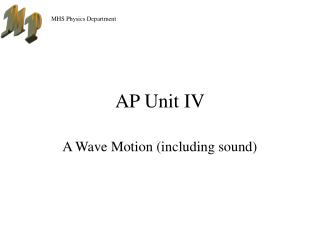AP Unit IV

# AP Unit IV - PowerPoint PPT Presentation

AP Unit IV A Wave Motion (including sound) 1. Traveling Waves Students should understand the description of traveling waves , so they can: (a) Sketch or identify graphs that represent traveling waves and determine the amplitude, wavelength, and frequency of a wave from such a graph.I am the owner, or an agent authorized to act on behalf of the owner, of the copyrighted work described.
Download Presentation## AP Unit IV

An Image/Link below is provided (as is) to download presentation

Download Policy: Content on the Website is provided to you AS IS for your information and personal use and may not be sold / licensed / shared on other websites without getting consent from its author.While downloading, if for some reason you are not able to download a presentation, the publisher may have deleted the file from their server.

- - - - - - - - - - - - - - - - - - - - - - - - - - E N D - - - - - - - - - - - - - - - - - - - - - - - - - -
Presentation Transcript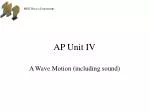### AP Unit IV

A Wave Motion (including sound)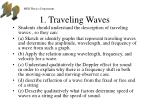1. Traveling Waves
• Students should understand the description of traveling waves , so they can:
• (a) Sketch or identify graphs that represent traveling waves and determine the amplitude, wavelength, and frequency of a wave from such a graph.
• (b) Apply the relation among wavelength, frequency, and velocity for a wave.
• (c) Understand qualitatively the Doppler effect for sound in order to explain why there is a frequency shift in both the moving-source and moving-observer case.
• (d) describe reflection of a wave from the fixed or free end of a string
• (e) Describe qualitatively what factors determine speed of waves on a string and the speed of sound.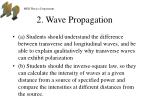2. Wave Propagation
• (a) Students should understand the difference between transverse and longitudinal waves, and be able to explain qualitatively why transverse waves can exhibit polarization
• (b) Students should the inverse-square law, so they can calculate the intensity of waves at a given distance from a source of specified power and compare the intensities at different distances from the source.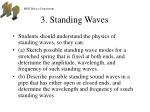3. Standing Waves
• Students should understand the physics of standing waves, so they can:
• (a) Sketch possible standing wave modes for a stretched spring that is fixed at both ends, and determine the amplitude, wavelength, and frequency of such standing waves.
• (b) Describe possible standing sound waves in a pipe that has either open or closed ends, and determine the wavelength and frequency of ssuch standing waves.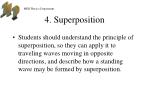4. Superposition
• Students should understand the principle of superposition, so they can apply it to traveling waves moving in opposite directions, and describe how a standing wave may be formed by superposition.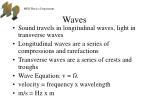Waves
• Sound travels in longitudinal waves, light in transverse waves
• Longitudinal waves are a series of compressions and rarefactions
• Transverse waves are a series of crests and troughs
• Wave Equation: v = fλ
• velocity = frequency x wavelength
• m/s = Hz x m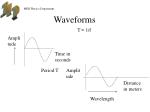Amplitude

Amplitude

Time in seconds

Distance in meters

Period T

Wavelength

Waveforms

T = 1/f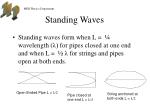Standing Waves
• Standing waves form when L = ¼ wavelength (λ) for pipes closed at one end and when L = ½ λ for strings and pipes open at both ends.

Open Ended Pipe L = λ/2

String anchored at both ends L = λ/2

Pipe closed at one end L = λ/4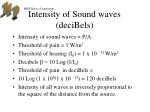Intensity of Sound waves (deciBels)
• Intensity of sound waves = P/A
• Threshold of pain = 1 W/m2
• Threshold of hearing (I0) = 1 x 10 –12 W/m2
• Decibels β = 10 Log (I/I0)
• Threshold of pain in deciBels =
• 10 Log (1 x 100/1 x 10 –12) = 120 deciBels
• Intensity of all waves is inversely proportional to the square of the distance from the source.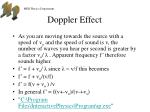Doppler Effect
• As you are moving towards the source with a speed of vo and the speed of sound is v, the number of waves you hear per second is greater by a factor vo/ λ . Apparent frequency f’ therefore sounds higher.
• f’ = f + vo/ λ since λ = v/f this becomes
• f’ = f + vo/(v/f)
• f’ = f(v + vo ) /v
• In general f’ = f(v+-vo)/(v-+vs)
• "C:\Program Files\InteractivePhysics\Program\ip.exe"Beats 14.11
• Superposition of two waves with slightly different frequencies. At times the waves are in phase and double in amplitude. At other times they are out of phase and cancel each other out. This causes different volumes and are heard as beats.The frequency f beat of the beats is equal to the difference in the two source frequencies f1 and f2
• f beat = f1 - f2